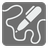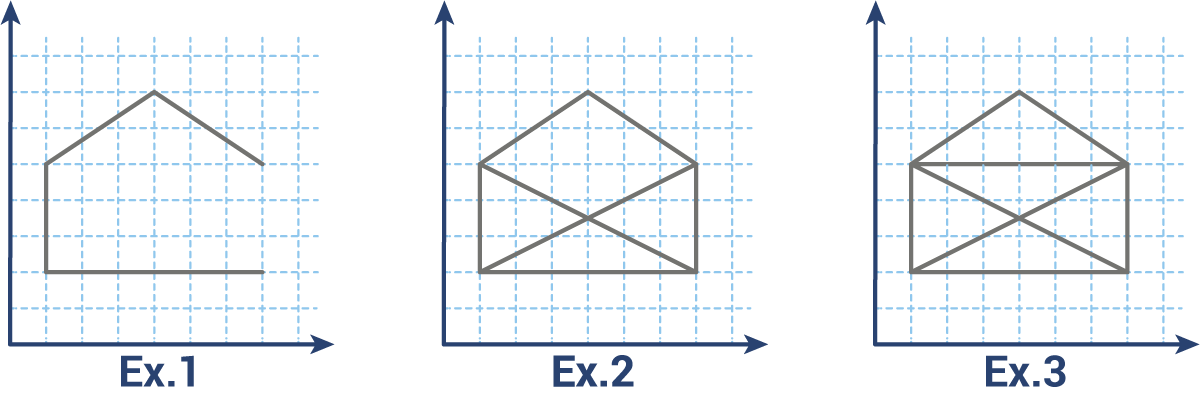One line Drawing
Simple
English RU FR

“The line that describes the beautiful is elliptical. It has simplicity and constant change. It cannot be described by a compass, and it changes direction at every one of its points.”

Rudolf Arnheim

Leonardo da Vinci, Raphael, Michelangelo, Albrecht Dürer, M.C. Escher, Hans Holbein, Paul Klee, LeRoy Neiman. All of these famous artists were left handed.

Our Robots have been spending some time researching the great artists from the Human civilization and would like to learn some of the fundamentals of art. For their first lesson, they need to learn to control their left hand-manipulators well enough to make smooth motions. For this exercise, they have decided to draw various figures on graph paper with an extra challenge rule -- don't lift your pen .

A figure is represented as a set of segments in the rectangular coordinate system. Each segment is represented as a sequence of 4 numbers: (x 1 , y 1 , x 2 , y 2 ), where x 1 , y 1 are coordinates for the first end and x 2 , y 2 -- for the second. Segments are undirected. All points in a figure are connected, so you can reach each point from any point.

You should find a path in order to draw the figure. You can pass through each segment only once and are not allowed to lift the pen. The result must be represented as a sequence of points (tuples with coordinates) in the order of how the pen moves to create the drawing. The path may be started and ended at any point. If it's impossible to draw a figure then return an empty sequence. Let's look at some examples:Example 1: the figure is represented as {(1,2,1,5),(1,2,7,2),(1,5,4,7),(4,7,7,5)} and can be two path - ((7,2),(1,2),(1,5),(4,7),(7,5)) or ((7,5),(4,7),(1,5),(1,2),(7,2)).
Example 2: the figure {(1,2,1,5),(1,2,7,2),(1,5,4,7),(4,7,7,5),(7,5,7,2),(1,5,7,2),(7,5,1,2)} can not be drawn with the given rules, so the result is an empty list or tuple.
Example 3: it's like fig.2 but with one more segment (1,5,7,5) and can be drawn several ways. One of them ((7,2),(1,2),(1,5),(4,7),(7,5),(7,2),(1,5),(7,5),(1,2)).

Input: Figure segments as a set of tuples with 4 integers each.

Output: The path as a list or tuple of tuples with 2 integers each.

Example:

```draw({(1,2,1,5),(1,2,7,2),(1,5,4,7),(4,7,7,5)}) == ((7,2),(1,2),(1,5),(4,7),(7,5))
draw({(1,2,1,5),(1,2,7,2),(1,5,4,7),(4,7,7,5),(7,5,7,2),(1,5,7,2),(7,5,1,2)}) == []
draw({(1,2,1,5),(1,2,7,2),(1,5,4,7),(4,7,7,5),(7,5,7,2),(1,5,7,2),(7,5,1,2),(1,5,7,5)}) == ((7,2),(1,2),(1,5),(4,7),(7,5),(7,2),(1,5),(7,5),(1,2))

```

How it is used: This mission illustrates the basics of computer generated vector graphics. The same principles may also be applied to AI pathfinding and topological work.

Precondition:
0 < len(segments) < 30
all(all(0 < x < 100 for x in s) for s in segments)

Settings
Code:
CheckiO Extensions

CheckiO Extensions allow you to use local files to solve missions. More info in a blog post.

In order to install CheckiO client you'll need installed Python (version at least 3.8)

Install CheckiO Client first:

`pip3 install checkio_client`

`checkio --domain=py config --key=`

Sync solutions into your local folder

`checkio sync`

(in beta testing) Launch local server so your browser can use it and sync solution between local file end extension on the fly. (doesn't work for safari)

`checkio serv -d`

Alternatevly, you can install Chrome extension or FF addon

`checkio install-plugin`
`checkio install-plugin --ff`
`checkio install-plugin --chromium`

Read more here about other functionality that the checkio client provides. Feel free to submit an issue in case of any difficulties.

Sync...